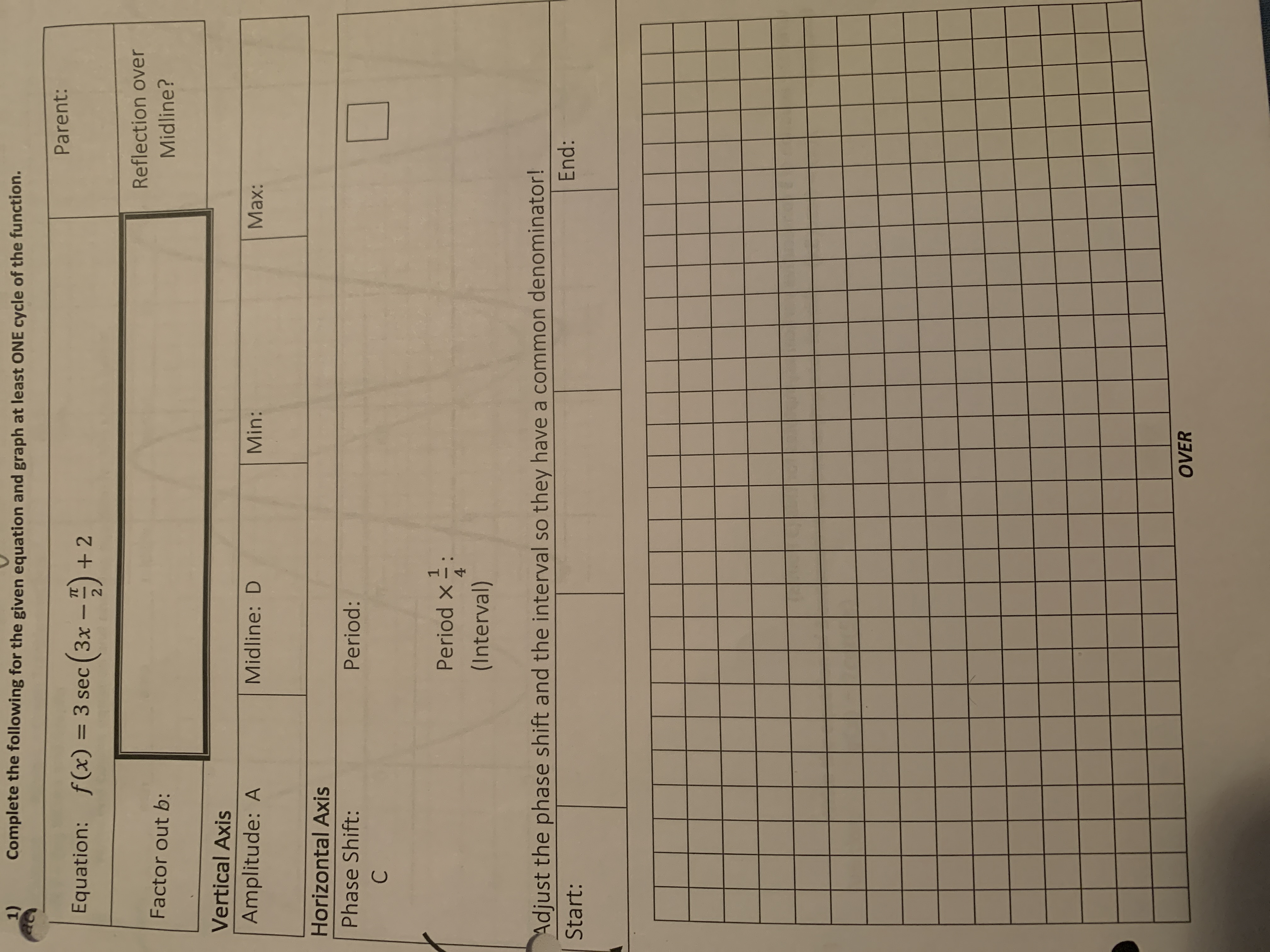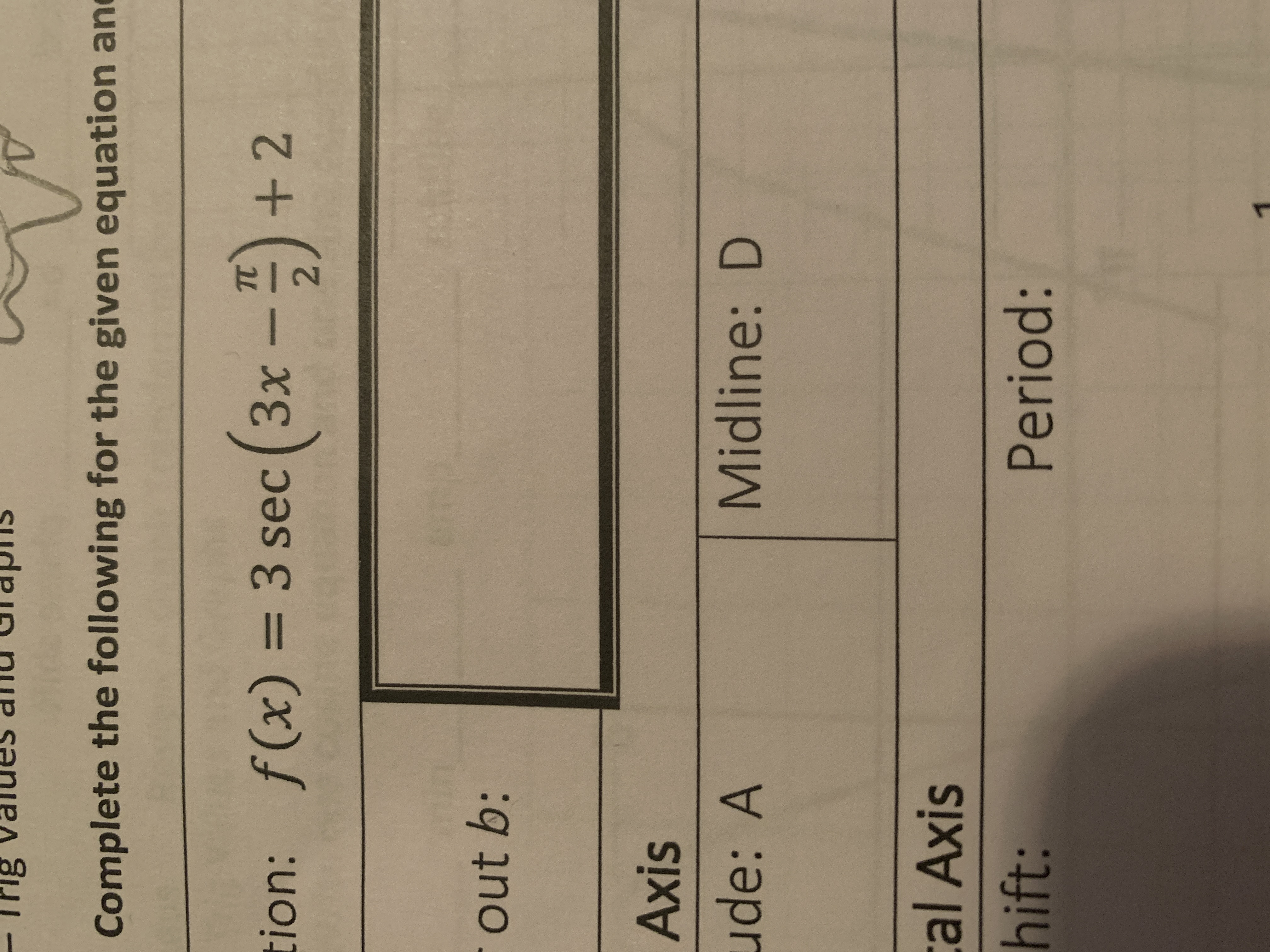# 1)Complete the following for the given equation and graph at least ONE cycle of the function.Parent:f(x) = 3 sec ( 3x - -).Equation:+ 2%3DReflection overFactor out b:Midline?Vertical AxisAmplitude: AMidline: DMin:Max:Horizontal AxisPhase Shift:Period:Period x = :4(Interval)Adjust the phase shift and the interval so they have a common denominator!End:Start:OVER Complete the following for the given equation anTC+ 2tion: f(x) = 3 sec (3x -)%3Dout b:Axisude: AMidline: Dcal Axishift:Period:

Question
29 views

What is the phase shift,min,max, and how do you graph, as well as factoring out b from the equation: f(x) = 3sec(3x-pi/2)+2help_outlineImage Transcriptionclose1) Complete the following for the given equation and graph at least ONE cycle of the function. Parent: f(x) = 3 sec ( 3x - -). Equation: + 2 %3D Reflection over Factor out b: Midline? Vertical Axis Amplitude: A Midline: D Min: Max: Horizontal Axis Phase Shift: Period: Period x = : 4 (Interval) Adjust the phase shift and the interval so they have a common denominator! End: Start: OVER fullscreenhelp_outlineImage TranscriptioncloseComplete the following for the given equation an TC + 2 tion: f(x) = 3 sec (3x -) %3D out b: Axis ude: A Midline: D cal Axis hift: Period: fullscreen
check_circle

Step 1

Given:

Step 2

Calculatio...

### Want to see the full answer?

See Solution

#### Want to see this answer and more?

Solutions are written by subject experts who are available 24/7. Questions are typically answered within 1 hour.*

See Solution
*Response times may vary by subject and question.
Tagged in

### Other